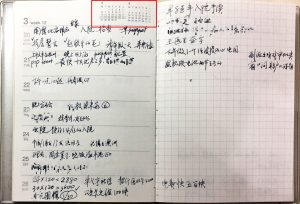# YMCA Song

Young man, there’s no need to feel down
I said, young man, pick yourself off the ground
I said, young man, ’cause you’re in a new town
There’s no need to be unhappy
Young man, there’s a place you can go
I said, young man, when you’re short on your dough
You can stay there, and I’m sure you will find
Many ways to have a good time
It’s fun to stay at the YMCA
It’s fun to stay at the YMCA
They have everything for you men to enjoy
You can hang out with all the boys
It’s fun to stay at the YMCA
It’s fun to stay at the YMCA
You can get yourself clean, you can have a good meal
You can do what ever you feel
Young man, are you listening to me?
I said, young man, what do you want to be?
I said, young man, you can make real your dreams
But you got to know this one thing
No man does it all by himself
I said, young man, put your pride on the shelf
And just go there, to the YMCA
It’s fun to stay at the YMCA
It’s fun to stay at the YMCA
They have everything for you men to enjoy
You can hang out with all the boys
It’s fun to stay at the YMCA
It’s fun to stay at the YMCA
You can get yourself clean, you can have a good meal
You can do what ever you feel
Young man, I was once in your shoes
I said, I was down and out with the blues
I felt no man cared if I were alive
I felt the whole world was so tight
That’s when someone came up to me
And said, young man, take a walk up the street
There’s a place there called the YMCA
They can start you back on your way
It’s fun to stay at the YMCA
It’s fun to stay at the YMCA
They have everything for you men to enjoy
You can hang out with all the boys
YMCA, it’s fun to stay at the YMCA
Young man, young man, there’s no need to feel down
Young man, young man, pick yourself off the ground
YMCA, it’s fun to stay at the YMCA
Young man, young man, are you listening to me
Young man, young man, what do you wanna be?
YMCA, you’ll find it at the YMCA
No man, young man, does it all by himself
Young man, young man, put your pride on the shelf
YMCA, and just go to the YMCA
Young man, young man I was once in your shoes
Young man, young man I was down with the blues, YMCA# 第67回NHK杯1回战第4局

133手 黑棋蘇耀国中盘胜

SGF 文件内容：

===========分割线，这行不算 ===============

(;CA[gb2312]AP[MultiGo:4.4.4]SZC[2019年04月28日 第67回NHK杯1回戦第4局

133手　黒\番　蘇耀国九段の中押し勝ち]
MULTIGOGM
;B[pd];W[cc];B[pp];W[cq];B[dd];W[dc];B[ed];W[cd];B[df];W[fc];B[dp];W[dq];B[ep];W[cp]
;B[dn];W[co];B[qn];W[do];B[fn];W[en];B[fm];W[em];B[el];W[fl];B[ek];W[fk];B[ej];W[fj]
;B[ei];W[hm];B[ho];W[jn];B[jp];W[kp];B[eo];W[dm];B[cm];W[cn];B[dl];W[dn];B[kq];W[lp]
;B[jo];W[kn];B[lq];W[mq];B[jq];W[mn];B[eq];W[qf];B[lm];W[mm];B[ll];W[mp];B[ln];W[ko]
;B[no];W[mo];B[jl];W[go];B[fo];W[hn];B[io];W[gp];B[gq];W[ik];B[ki];W[nk];B[il];W[hl]
;B[ii];W[gh];B[in];W[im];B[jm];W[hq];B[gr];W[ij];B[ni];W[pk];B[pi];W[pr];B[qq];W[qr]
;B[hi];W[gi];B[hj];W[hk];B[ge];W[hg];B[ol];W[ok];B[rk];W[oh];B[oi];W[mj];B[jj];W[jk]
;B[lj];W[mi];B[jh];W[jf];B[kf];W[lh];B[kg];W[he];B[hd];W[id];B[hf];W[if];B[gf];W[kk]
;B[lk];W[ig];B[nh];W[mf];B[kd];W[md];B[nl];W[ml];B[mg];W[kj];B[li];W[mh];B[lg];W[kh]
;B[ji];W[jg];B[mk];W[nj];B[pl];W[qk];B[rj])

===========以上，这行不算 =================# 第67回NHK杯1回战第3局

297手 白棋林漢傑2目半胜

SGF 文件内容：

===========分割线，这行不算 ===============

(;CA[gb2312]AP[MultiGo:4.4.4]SZC[2019年04月21日 第67回NHK杯1回戦第3局

297手　白番　林漢傑八段の2目半勝ち]
MULTIGOGM
;B[qd];W[dp];B[dc];W[ce];B[pp];W[ed];B[ec];W[fd];B[gc];W[oc];B[pc];W[od];B[qf];W[qq]
;B[pq];W[qp];B[qo];W[ro];B[rn];W[qn];B[po];W[rm];B[rp];W[sn];B[rq];W[gd];B[hc];W[hd]
;B[ic];W[dj];B[cq];W[cp];B[dq];W[ep];B[fr];W[fq];B[gr];W[ho];B[id];W[ie];B[je];W[if]
;B[jf];W[ig];B[jg];W[ih];B[ki];W[kd];B[md];W[lf];B[mb];W[og];B[oi];W[me];B[ld];W[ke]
;B[jd];W[pe];B[qe];W[ob];B[kc];W[ph];B[qj];W[qi];B[rj];W[pj];B[pi];W[qh];B[oh];W[pf]
;B[ql];W[pk];B[rl];W[pl];B[qm];W[pm];B[pn];W[qb];B[rb];W[ra];B[pb];W[pa];B[qc];W[qa]
;B[rg];W[ri];B[sb];W[nj];B[mh];W[mi];B[ni];W[oj];B[nh];W[qk];B[rk];W[sj];B[rn];W[rc]
;B[rd];W[qn];B[qg];W[pg];B[rn];W[lh];B[li];W[qn];B[cj];W[ck];B[rn];W[mj];B[lg];W[qn]
;B[bk];W[rn];B[ci];W[bl];B[sp];W[sk];B[cf];W[dk];B[be];W[cd];B[bd];W[df];B[cg];W[cc]
;B[eh];W[bq];B[gq];W[cr];B[jq];W[qr];B[pr];W[bj];B[bi];W[ak];B[ef];W[ff];B[dr];W[br]
;B[jo];W[lo];B[go];W[ip];B[hp];W[io];B[iq];W[rr];B[sr];W[bc];B[dg];W[fg];B[di];W[fo]
;B[fp];W[fc];B[fb];W[gp];B[mn];W[ln];B[fp];W[eq];B[lm];W[kn];B[fn];W[eo];B[jn];W[km]
;B[jm];W[ll];B[kl];W[mm];B[gl];W[hk];B[hl];W[il];B[ik];W[jl];B[dm];W[er];B[cn];W[cm]
;B[bm];W[en];B[em];W[cl];B[dn];W[bn];B[mp];W[lp];B[lq];W[mq];B[mr];W[mo];B[nq];W[gk]
;B[fk];W[ij];B[bo];W[am];B[cb];W[bb];B[gm];W[jk];B[ca];W[fj];B[gp];W[db];B[eb];W[co]
;B[nn];W[no];B[om];W[mg];B[kh];W[ng];B[lh];W[ba];B[da];W[fl];B[fh];W[gh];B[gi];W[eg]
;B[de];W[dd];B[fi];W[hi];B[gg];W[hh];B[fe];W[gf];B[ge];W[hg];B[ee];W[he];B[df];W[nd]
;B[ol];W[mc];B[lc];W[ai];B[ah];W[aj];B[bg];W[el];B[fm];W[nl];B[lj];W[lk];B[nc];W[nb]
;B[np];W[oo];B[on];W[ma];B[la];W[na];B[mc];W[kp];B[kf];W[le];B[pd];W[ne];B[kq];W[op]
;B[oq];W[fs];B[gs];W[es];B[ok];W[nk];B[sh];W[so];B[rs];W[gj];B[jh];W[ac];B[ae];W[rh]
;B[ei];W[kk];B[ji])

===========以上，这行不算 =================# 第67回NHK杯1回战第2局

136手　白棋六浦雄太中盘胜

SGF 文件内容：

===========分割线，这行不算 ===============

(;CA[gb2312]AP[MultiGo:4.4.4]SZC[2019年04月14日 第67回NHK杯1回戦第2局

136手　白棋中盘胜]
MULTIGOGM
;B[qd];W[dp];B[dc];W[pp];B[cn];W[ce];B[dg];W[ee];B[fd];W[di];B[fg];W[cg];B[fq];W[fp]
;B[gp];W[fo];B[cq];W[eq];B[cp];W[gq];B[ck];W[df];B[kc];W[pd];B[qe];W[pc];B[qc];W[qb]
;B[pe];W[nd];B[oe];W[lc];B[lb];W[mc];B[kd];W[rb];B[qn];W[ql];B[on];W[np];B[qj];W[pj]
;B[qk];W[pk];B[rl];W[pl];B[rm];W[ph];B[pi];W[oi];B[qi];W[nh];B[og];W[oh];B[rg];W[gf]
;B[gd];W[ie];B[ic];W[kp];B[nj];W[nl];B[mn];W[ll];B[lj];W[lh];B[jj];W[jh];B[il];W[km]
;B[in];W[jo];B[jn];W[kn];B[hq];W[fr];B[io];W[hr];B[hi];W[ip];B[lo];W[ko];B[oq];W[op]
;B[pq];W[nq];B[qq];W[qp];B[nr];W[mr];B[or];W[hk];B[ik];W[hj];B[ii];W[dk];B[fk];W[cl]
;B[ei];W[ej];B[fj];W[eh];B[fi];W[bo];B[co];W[bn];B[cm];W[bm];B[dl];W[bl];B[ek];W[dj]
;B[em];W[oj];B[mq];W[mp];B[cd];W[bd];B[bc];W[lq];B[rp];W[ro];B[rq];W[gn];B[gm];W[qo]

===========以上，这行不算 =================# 第67回NHK杯1回战第1局

190手白棋孫喆七段中盘胜

SGF 文件内容：

===========分割线，这行不算 =================

(;CA[gb2312]AP[MultiGo:4.4.4]SZC[2019年04月07日 第67回NHK杯1回戦第1局

190手白棋孫喆七段中盘胜]
MULTIGOGM
;B[qd];W[dp];B[cd];W[pp];B[oc];W[ec];B[df];W[id];B[qn];W[nq];B[cn];W[co];B[dn];W[fp]
;B[cj];W[pj];B[qp];W[qq];B[rq];W[qo];B[rp];W[ro];B[po];W[rn];B[pq];W[op];B[pr];W[qm]
;B[hq];W[er];B[mp];W[kp];B[gn];W[mq];B[jo];W[dj];B[ck];W[dh];B[ko];W[ci];B[cg];W[bj]
;B[bk];W[gi];B[ei];W[di];B[bi];W[bh];B[aj];W[cc];B[bc];W[dd];B[cb];W[ce];B[dc];W[bd]
;B[cc];W[de];B[fc];W[eb];B[ed];W[ee];B[fd];W[fe];B[fb];W[bg];B[qg];W[jp];B[ip];W[lo]
;B[ln];W[lp];B[km];W[mc];B[pn];W[pm];B[on];W[mn];B[mm];W[nn];B[om];W[nm];B[ol];W[pk]
;B[nk];W[ml];B[mk];W[ri];B[qi];W[qj];B[ll];W[lm];B[rk];W[rl];B[mm];W[nl];B[ok];W[lm]
;B[rj];W[rh];B[qh];W[rg];B[rf];W[qk];B[mm];W[lk];B[lj];W[kk];B[kl];W[jk];B[ki];W[ji]
;B[jh];W[ii];B[kh];W[pe];B[sg];W[sk];B[oh];W[qf];B[re];W[od];B[pc];W[mf];B[ng];W[mg]
;B[mh];W[pg];B[ph];W[nf];B[je];W[jd];B[ke];W[ld];B[of];W[pf];B[og];W[oe];B[lg];W[lf]
;B[kf];W[ni];B[oi];W[oj];B[nj];W[mi];B[nh];W[pi];B[lh];W[ig];B[jg];W[if];B[ih];W[hh]
;B[ie];W[gd];B[he];W[gb];B[ea];W[gc];B[db];W[gf];B[le];W[me];B[kd];W[kc];B[lb];W[lc]
;B[nc];W[nd];B[mb];W[ib];B[jf];W[jr];B[jq];W[kq];B[iq];W[lr];B[hj];W[hk];B[hg];W[gj]
;B[il];W[jl];B[ik];W[jm];B[in];W[ho];B[im];W[jn])

===========以上，这行不算 =================# 清明无锡游记录# 作为一个经验丰富的团队怎么办？# 卡桑德拉

1.卡珊德拉在阿波罗神庙玩耍，玩累便睡着了。阿波罗试图拥抱卡珊德拉却遭到反抗，遂使她的预言不被相信，见于许癸努斯（Hyginus）的《神话指南》（Fabulae）。

2.阿波罗多洛斯（Apollodorus）《书库》（The library）提及“阿波隆（阿波罗其它译名）想要与卡珊德拉交会，应许教给她占卜术，她学会了，可是不和他交会，于是阿波隆夺去了她占卜的使人信用的力量。”（周作人译）

3.埃斯库罗斯阿伽门农》，依照罗念生译本，卡珊德拉允诺委身阿波罗却又使他失望，此后再也没有人相信她。但在其他译本，“他是个摔角手，恩情往我身上喷。”（吕健忠译）“他将我扭抱，把我摔倒，吐喘甜蜜的欲火”（陈中梅译）“他与我扭斗，同时他兴奋喘息。”（刘毓秀译）似曾有肌肤之亲，后文提及决裂的原因是因为卡珊德拉承诺要为他生下子嗣却出言不果。中研院研究员李奭学先生〈长夜后的黎明－－试论《奥勒斯提亚》的一则主题故事〉一文以圣婚概念解释剧中阿波罗与卡珊德拉间的纠葛。# 2017年效率工具回顾

（一）整个2017年我都在用一本无印良品的月周历本，A6的。2018年，我买了一本B6的，红封面的。想保持自己记录生活的好习惯。

（二）在工作上，我多多的使用了Trello这个工具。

2017下半年以后我基本在实践一种给每周建立一个列表，

Trello又是全平台的，在安卓和iOS上都有App，可以随时用起来。

2018年我会继续实践这种看板工作流。

（三）在头脑风暴方面，我还是喜欢在电脑上用XMind来画脑图。

（四）最后，最好的效率工具永远是纸笔。多写，多记，多画。